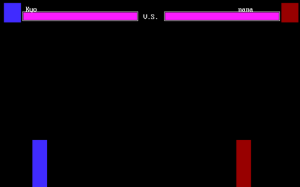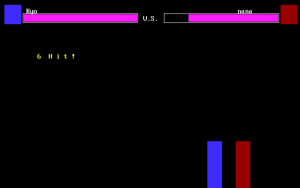96年QBASIC练习程序。FOR I = 1 TO 50000: NEXT I COLOR 14: LOCATE 10, 35: PRINT “V . S .”: FOR I = 1 TO 70000: NEXT I COLOR 0: LOCATE 10, 35: PRINT “V . S .”

DO IF h1 = 1 GOTO 310 I\$ = INKEY\$ SELECT CASE I\$

CASE “w” IF j1 = 0 THEN j1 = 1 CASE “s” CASE “a” IF x1 > 5 AND NOT righting = 1 THEN left = 1 CASE “d” IF x1 + 40 < x2 AND NOT lefting = 1 THEN right = 1 CASE “h” h1 = 1 END SELECT 310 IF h2 = 1 GOTO 400 400 IF j1 = 1 THEN IF left = 1 THEN lefting = 1: jx = -2 IF right = 1 THEN righting = 1: jx = 2 IF y1 = 149 THEN n1 = 1 IF n1 = 1 THEN IF y1 < 299 THEN y1 = y1 + 5 IF x1 > 5 AND (y1 + 100 < y2 OR (y1 + 100 > y2 AND (x1 + 40 < x2 OR x1 > x2 + 40))) THEN x1 = x1 + jx ELSE j1 = 0: n1 = 0 END IF ELSE y1 = y1 - 5 IF x1 > 5 AND (y1 + 100 < y2 OR (y1 + 100 > y2 AND (x1 + 40 < x2 OR x1 > x2 + 40))) THEN x1 = x1 + jx END IF ELSE lefting = 0: righting = 0: jx = 0 END IF IF left = 1 AND j1 = 0 THEN x1 = x1 - 10 IF right = 1 AND j1 = 0 THEN x1 = x1 + 10 IF j2 = 1 THEN GOSUB 20000 IF l1 = 1 OR l2 = 1 THEN GOSUB 80000 PUT (x1, y1), a%, OR IF S1 = 0 THEN GOTO 90000 FOR I = 1 TO 1000: NEXT I PUT (x1, y1), a%, PSET IF h1 = 1 THEN i1 = x1 + 30: k1 = y1 + 10 GET (i1, k1)-(i1 + 50, k1 + 10), c% LINE (i1, k1)-(i1 + 50, k1 + 10), 9, BF FOR a = 1 TO 3000: NEXT a PUT (i1, k1), c%, PSET GOSUB 30000 END IF FOR t = 1 TO 1000: NEXT t PUT (x1, y1), b%, PSET left = 0: right = 0 LOOP 90000
COLOR 13: LOCATE 10, 36: PRINT “K . O .”: FOR I = 1 TO 100000: NEXT I COLOR 0: LOCATE 10, 36: PRINT “K . O .” IF S1 = 0 THEN WIN\$ = P1\$: IF S2 = 0 THEN WIN\$ = P2\$: COLOR 14 LOCATE 10, 36: PRINT WIN\$ LOCATE 12, 36: PRINT “W I N !” I\$ = INPUT\$(1) COLOR 0 END 20000 RETURN 30000 IF i1 + 50 >= x2 AND k1 + 5 >= y2 AND k1 <= y2 + 100 THEN t1 = t1 + 1 IF t1 >= 2 THEN COLOR 14 LOCATE 8, 10 PRINT t1; “ H i t !” COLOR 0 l2 = 1: h1 = 0 END IF ELSE h1 = 0 END IF RETURN 40000 RETURN 80000 FOR I = 1 TO 5000: NEXT I IF l1 = 1 THEN IF S1 - 10 < 0 THEN S1 = 0 ELSE S1 = S1 - 10 END IF END IF LINE (291, 30)-(51 + S2, 45), 0, BF IF l2 = 1 THEN IF S1 - 10 < 0 THEN S1 = 0 ELSE S1 = S1 - 10 END IF END IF LINE (349, 30)-(589 - S1, 45), 0, BF l1 = 0: l2 = 0 RETURN

</code>